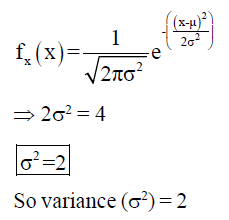Courses

# Communication System

## 26 Questions MCQ Test GATE ECE (Electronics) 2022 Mock Test Series | Communication System

Description
This mock test of Communication System for Electronics and Communication Engineering (ECE) helps you for every Electronics and Communication Engineering (ECE) entrance exam. This contains 26 Multiple Choice Questions for Electronics and Communication Engineering (ECE) Communication System (mcq) to study with solutions a complete question bank. The solved questions answers in this Communication System quiz give you a good mix of easy questions and tough questions. Electronics and Communication Engineering (ECE) students definitely take this Communication System exercise for a better result in the exam. You can find other Communication System extra questions, long questions & short questions for Electronics and Communication Engineering (ECE) on EduRev as well by searching above.
QUESTION: 1

### The DSB-SC signal recevied at the output of a channel is given as S(t) = 2 cos2π(103 - 0.5). cos2π(106 - 1). Determine the group delay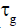and phase delay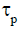introduced by the channel.

Solution:

For an amplitude modulated signal
the delay in carrier frequency
component gives phase delay and
delay in baseband (message)
signal gives the group delay.
{Reference: Page No 98, Simon
Haykin, common system 3rd
edition}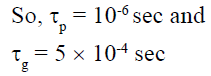QUESTION: 2

### Which of the following is incorrect about hilbert-transform?

Solution:

xh (t) is delayed version by -900 of
x(t)

QUESTION: 3

### An amplitude modulated signal is given as s(t) = 14 cos8000πt + 20 cos10000πt + 14 cos12000πt. Determine the modulation depth.

Solution: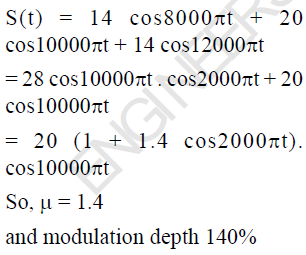QUESTION: 4

An amplitude modulated signal is given as S(t) = 10 (2 + cos2π x 104t) cos2π x 107t. If the carrier
frequency component is suppresed before transmission, then determine the percentage power
saved.

Solution: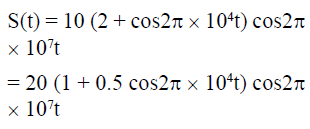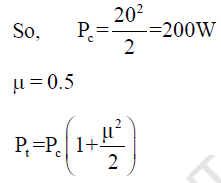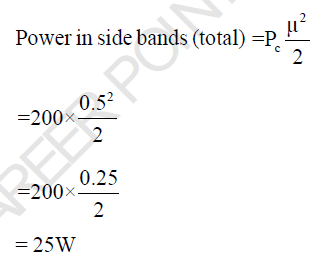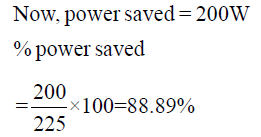QUESTION: 5

Suppose that non linear device (NLD) is available for which the output voltage V0 and input voltage Vi are related by V0(t) = a1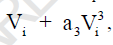where a1 and a3 are constants. Let Vi = m (t) + cos2πfct and a1 = 1, a2 = 1. To generate a DSB-SC signal having carrier frequency is 5MHz, this NLD is followed by a BPF of appropriate centre frequency and band width. Determine fc

Solution: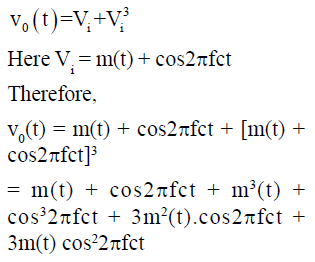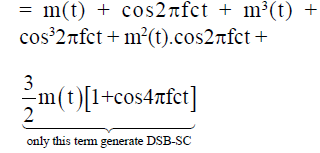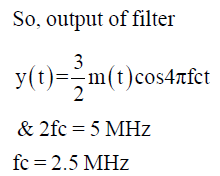QUESTION: 6

Consider a square law device having input voltage Vi and output voltage V0 are related as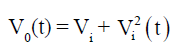This SLD is used to generate an standard AM with a carrier frequency of 3MHz, as shown in the circuit below.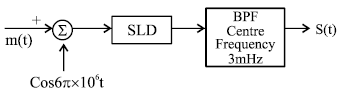Determine the maximum bandwidth of message signal m(t) so that spectrum of s(t) is nonoverlapping.

Solution: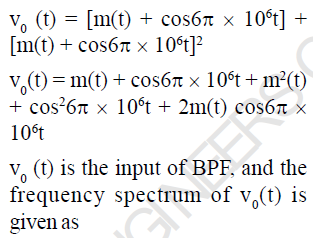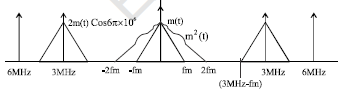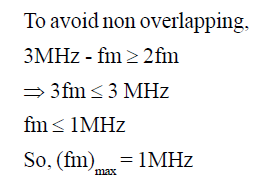i.e the maximum bandwidth of
message signal may be 1MHz

QUESTION: 7

A message signal m(t) = 4 cos2πx 104 t is used to generate an FM signal. Determine the minimum
value of (Δf)max so that modulation efficiency is 100%.

Solution:

M(t) = 4cos2π x 104t
Here Am = 4, fm = 10 KHz,
Modulation efficiency is 100%

when βf = 2.4, 5.5, 8.6-----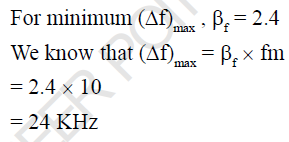QUESTION: 8

A phase-modulated signal is given as S(t) = 30 cos[2πx108t + 100 cos2πx104t]. Determine the
transmission bandwidth required according to carson’s approximation.

Solution: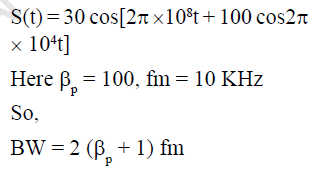= 2 (100 + 1) x 10
= 20 x 101
= 2020 KHz
= 2.02 MHz

QUESTION: 9

Consider an FM signal, S(t) = 10 cos[2π x107t + 10 sin2π x103t]. Determine Kp/Kf if the above modulated signal is assumed phase-modulated signal.

Solution: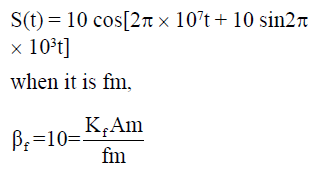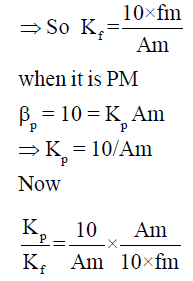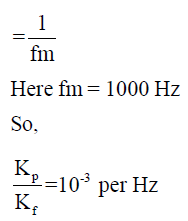QUESTION: 10

The following message signal m(t) is given to both frequency modulator and phase modulator. If
the maximum phase deviation of both FM and PM is same, determine the Kf/Kp.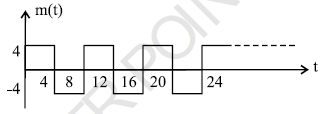Solution: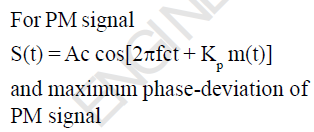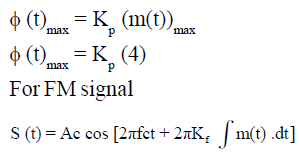and maximum phase-deviation of
FM signal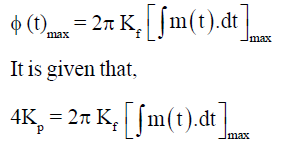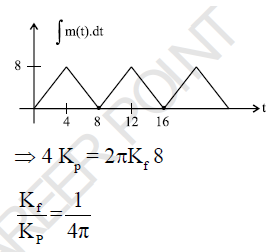QUESTION: 11

Consider a sine wave of frequency 2 KHz and peak amplitude AM. This signal is applied to a delta modulator of step-size 0.4 Volt and sampling period 3.18 x 10-6 sec. What is the maximum power that
may be transmitted without slopeoverload distortion?

Solution: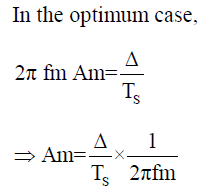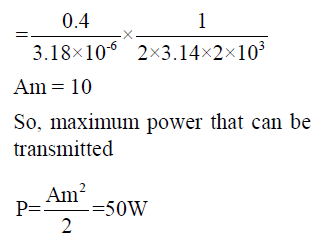QUESTION: 12

A PCM system uses an uniform quantizer followed by a 7-bit binary encoder. The bit rate of the system is equal to 50x106 bits/ second. Determine the output signal to quantization noise ratio when a full-load sinusoidal modulating signal of frequency 1MHz is applied to the input.

Solution:

(SNR) = (6n + 1.8) dB
= 6 x 7 + 1.8
= 43.5 dB

QUESTION: 13

An analog signal is sampled, quantized and encoded into a binary PCM system. The specifications of the PCM system include the following: Sampling role = 8 KHz Number of representation levels = 64
Determine the minimum bandwidth required if PCM wave is transmitted over a baseband channel.

Solution: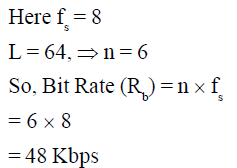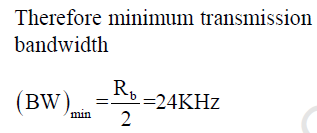QUESTION: 14

A binary PCM system uses pulse P(t) to transmit symbol 1 and - P(t) to transmit symbol 0. The additive noise at the reciever input is white and Gaussian, with zero mean and power spectral density
10-10 W/Hz. Assuming that symbols 1 and 0 occur with equal probability, determine the probability of error at the reciever output.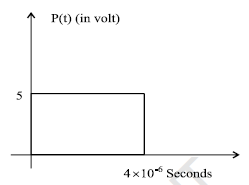Solution:

For binary polar signal the
probability of error is given as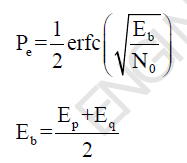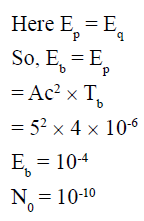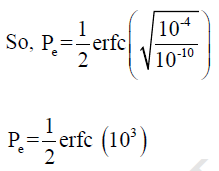QUESTION: 15

A source emits one of four symbols S0, S1, S2 and S3 with probabilities 1/3, 1/6, 1/4 and 1/4 respectively.
The successive symbols emitted by the source are statistically independent. Calculate the entropy
of the source.

Solution: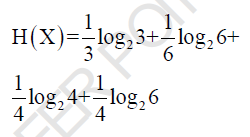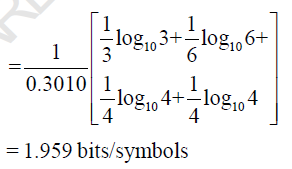QUESTION: 16

Consider a sinusoidal signal with random phase, defined by X(t) =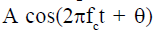where A and fare constants and θ is a random variable that is uniformly distributed over the interval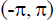Determine the autocorelation function of X (t)

Solution:

The probability function of random
variable is given as,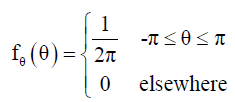Now the auto-corelation function
of X(t)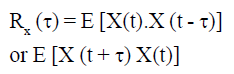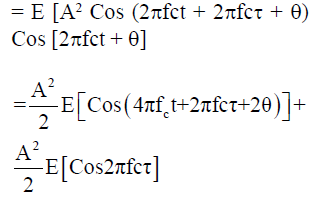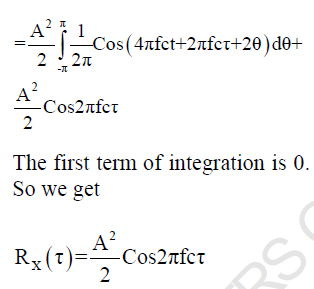QUESTION: 17

The probability density function of a random variable is shown in the figure. Determine P (X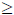0)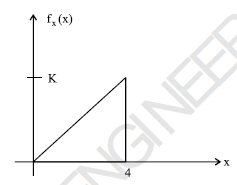Solution:

The probability for X0 is exactly
1. (i.e whole area under density
function curve)

QUESTION: 18

A 1W video signal having bandwidth of 200 MHz is transmitted to a reciver through a cable that has 50 dB loss. If the efficitive two sided noise power spectred density at the receiver is 10-20 Watt/Hz, then the signal to noise ratio at the receiver is

Solution:

PS = 1 W
Since cable loss is 50 dB (105), So
power at the input of reciever
PS = 10-5 Watts
Noise power = 2 x200 x106 x10-20
2, Because of two sided PSD
= 4 x 10-12 Watts
Therefore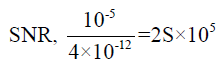QUESTION: 19

A computer puts out binary data at the rate of 56 kbps. The computer output is transmitted using a
baseband binary PAM system that is designed to have a raised cosine spectrum. Determine band width
required if the roll-off factor is 0.5.

Solution:

Bit Rate (Rb) = 56 Kbps
Since it is transmitted by 2-array,
so minimum bandwidth without
raised-cosine pattern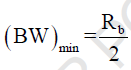and minimum bandwidth with
raised-cosine pattern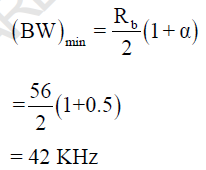QUESTION: 20

A QPSK system transmits binary data at the rate of 2.5 x 106 bit per second. Determine minimum
bandwidth required for the transmission.

Solution: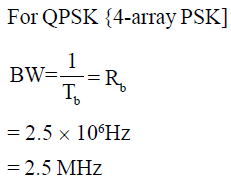QUESTION: 21

For attenuation of high frequencies we should use

Solution:

Since Xc for high frequencies is low, the high frequencies are shunted to ground and are not transmitted.

QUESTION: 22

The pulse shown in the figure, is given as the input of a matched filter. Determine the peak value of
the output of matched filter.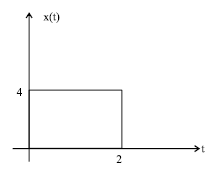Solution: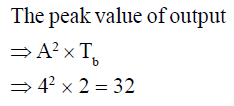QUESTION: 23

A binary random variable X takes the value of 1 with probability 2/3. X is input to a binary symetric
channel with cross-over probability 1/2.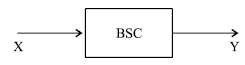Determine the value of H(Y)

Solution: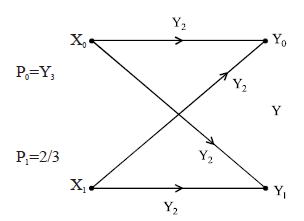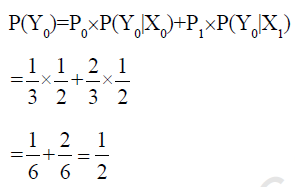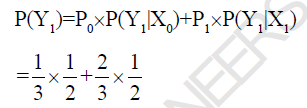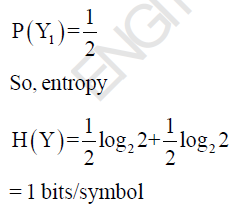QUESTION: 24

A voice-grade channel of the telephone network has a bandwidth of 3.4 KHz calculate the information capacity of the telephone channel for a signal to noise ratio of 30 dB.

Solution:

Capacity of a gaussian channel is
given as,

C = BW log2 (1 + SNR)

Here SNR = 30 dB (1000)
So,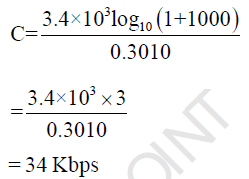QUESTION: 25

Determine the minimum sampling frequency of x(2t), where x(t) = sine (1000t)

Solution:

Minimum sampling frequency of
x(t) is 1000 Hz (1KHz)
So, minimum sampling frequency
of x(2t) is 2KHz

QUESTION: 26

The probability density function of random variable X is given as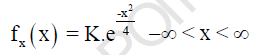Determine the variance (σ2) of random variable X.

Solution:

Given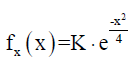Comparing this equation with the
standard gaussian probability
density function
i.e